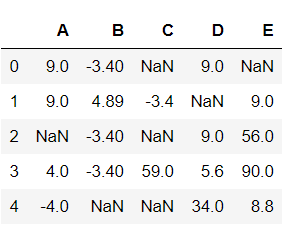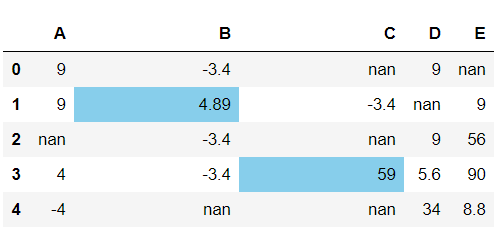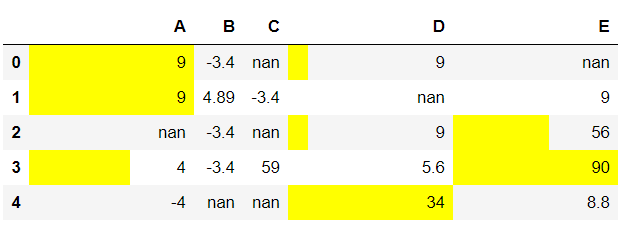# How to display bar charts in Pandas dataframe on specified columns?

In this article we will see how to display bar charts in dataframe on specified columns. For doing this task we are using DataFrame.style.bar() method of Pandas Dataframe.

Syntax: pandas.DataFrame.style.bar(columns_list, color)

Return: Dataframe with the given color bar strips on the positive definite values. The None value and the negative values are skipped in the process.

Now, Let’s create a Dataframe:

## Python3

 `# importing required packages ` `import` `pandas ` `import` `numpy ` ` `  `Nan ``=` `numpy.nan ` ` `  `# creating a dataframe with some nan values ` `df ``=` `pandas.DataFrame(data ``=` `numpy.array( ` `                      ``[[``9``, ``-``3.4``, Nan, ``9``, Nan], ` `                       ``[``9``, ``4.89``, ``-``3.4``, Nan, ``9``], ` `                       ``[Nan, ``-``3.4``, Nan, ``9``, ``56``], ` `                       ``[``4``, ``-``3.4``, ``59.0``, ``5.6``, ``90``], ` `                       ``[``-``4``, Nan, Nan, ``34``, ``8.8``]]), ` `                       ``columns ``=` `list``(``"ABCDE"``)) ` ` `  `# view dataframe ` `df`

Output:Example 1:

## Python3

 `# display the bar chart on dataframe ` `df.style.bar(subset ``=` `[``'B'``, ``'C'``], ` `                   ``color ``=` `'skyblue'``)`

Output:Example 2:

## Python3

 `# display the bar chart on dataframe ` `df.style.bar(subset ``=` `[``'A'``, ``'D'``, ``'E'``], ` `                   ``color ``=` `'yellow'``)`

Output:Attention geek! Strengthen your foundations with the Python Programming Foundation Course and learn the basics.

To begin with, your interview preparations Enhance your Data Structures concepts with the Python DS Course.

My Personal Notes arrow_drop_upCheck out this Author's contributed articles.

If you like GeeksforGeeks and would like to contribute, you can also write an article using contribute.geeksforgeeks.org or mail your article to contribute@geeksforgeeks.org. See your article appearing on the GeeksforGeeks main page and help other Geeks.

Please Improve this article if you find anything incorrect by clicking on the "Improve Article" button below.

Article Tags :

Be the First to upvote.

Please write to us at contribute@geeksforgeeks.org to report any issue with the above content.